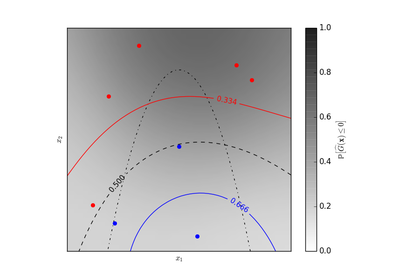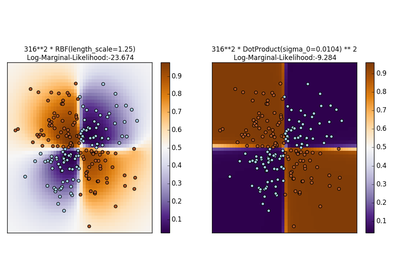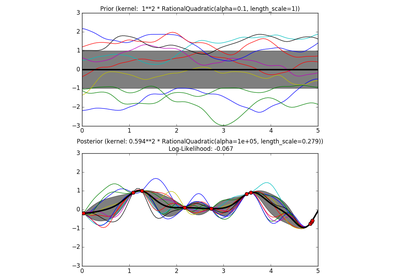# `sklearn.gaussian_process.kernels`.DotProduct¶

class `sklearn.gaussian_process.kernels.``DotProduct`(sigma_0=1.0, sigma_0_bounds=(1e-05, 100000.0))[source]

Dot-Product kernel.

The DotProduct kernel is non-stationary and can be obtained from linear regression by putting N(0, 1) priors on the coefficients of x_d (d = 1, . . . , D) and a prior of N(0, sigma_0^2) on the bias. The DotProduct kernel is invariant to a rotation of the coordinates about the origin, but not translations. It is parameterized by a parameter sigma_0^2. For sigma_0^2 =0, the kernel is called the homogeneous linear kernel, otherwise it is inhomogeneous. The kernel is given by

k(x_i, x_j) = sigma_0 ^ 2 + x_i cdot x_j

The DotProduct kernel is commonly combined with exponentiation.

New in version 0.18.

Parameters: sigma_0 : float >= 0, default: 1.0 Parameter controlling the inhomogenity of the kernel. If sigma_0=0, the kernel is homogenous. sigma_0_bounds : pair of floats >= 0, default: (1e-5, 1e5) The lower and upper bound on l

Methods

 `clone_with_theta`(theta) Returns a clone of self with given hyperparameters theta. `diag`(X) Returns the diagonal of the kernel k(X, X). `get_params`([deep]) Get parameters of this kernel. `is_stationary`() Returns whether the kernel is stationary. `set_params`(\*\*params) Set the parameters of this kernel.
`__init__`(sigma_0=1.0, sigma_0_bounds=(1e-05, 100000.0))[source]
`bounds`

Returns the log-transformed bounds on the theta.

Returns: bounds : array, shape (n_dims, 2) The log-transformed bounds on the kernel’s hyperparameters theta
`clone_with_theta`(theta)[source]

Returns a clone of self with given hyperparameters theta.

`diag`(X)[source]

Returns the diagonal of the kernel k(X, X).

The result of this method is identical to np.diag(self(X)); however, it can be evaluated more efficiently since only the diagonal is evaluated.

Parameters: X : array, shape (n_samples_X, n_features) Left argument of the returned kernel k(X, Y) K_diag : array, shape (n_samples_X,) Diagonal of kernel k(X, X)
`get_params`(deep=True)[source]

Get parameters of this kernel.

Parameters: deep: boolean, optional : If True, will return the parameters for this estimator and contained subobjects that are estimators. params : mapping of string to any Parameter names mapped to their values.
`hyperparameters`

Returns a list of all hyperparameter specifications.

`is_stationary`()[source]

Returns whether the kernel is stationary.

`n_dims`

Returns the number of non-fixed hyperparameters of the kernel.

`set_params`(**params)[source]

Set the parameters of this kernel.

The method works on simple kernels as well as on nested kernels. The latter have parameters of the form `<component>__<parameter>` so that it’s possible to update each component of a nested object.

Returns: self :
`theta`

Returns the (flattened, log-transformed) non-fixed hyperparameters.

Note that theta are typically the log-transformed values of the kernel’s hyperparameters as this representation of the search space is more amenable for hyperparameter search, as hyperparameters like length-scales naturally live on a log-scale.

Returns: theta : array, shape (n_dims,) The non-fixed, log-transformed hyperparameters of the kernel

## Examples using `sklearn.gaussian_process.kernels.DotProduct`¶Iso-probability lines for Gaussian Processes classification (GPC)Illustration of Gaussian process classification (GPC) on the XOR datasetIllustration of prior and posterior Gaussian process for different kernels